/////////////////////////////////////////////////////////////\\\\\\\\\\\\\\\\\\\\\\\\\\\\\\\\\\\\\\\\\\\\\\\\Dedication Time consistencY

 σy↑⇀τyx

σx←τxy

# Structural Analysis

Structural Analysis is the forecast of the performance of a structure under prescribed loading conditions. The results that are of interest are: the deflections, reactions and stresses.

Equations:

• {Q} = {Qf}+ [k]{u}---->At the member level (local)

• where:
{Q} = Member forces
{Qf}= Fixed-end member forces
[k] = member stiffness matrix
{u} = member displacements

• {P} = {Pf}+ [S]{d}---->At the structural level (global)

• where:
{P} = Joint forces
{Pf}= Fixed-end forces
[S] = stiffness matrix
{d} = displacements

## Displacement, Reaction and Member Forces in an Axial Structure (Matrix Displacement Method,MDM)

1. Degrees of Freedom (DOF) for an axial member
2.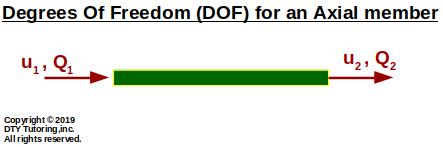3. Finding displacement, reaction and member forces using MDM method example (Axial)
4. Find the displacements, member forces and reactions for this axial structure. Then, draw the axial diagram.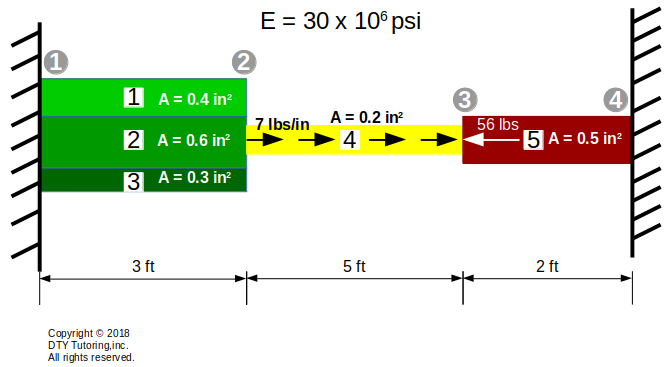StructuralAnalysisAxialEx2solution.pdf

5. Finding displacement, reaction and member forces using MDM method example (Axial)
6. Find the displacements, member forces and reactions for this axial structure. Then, draw the axial diagram.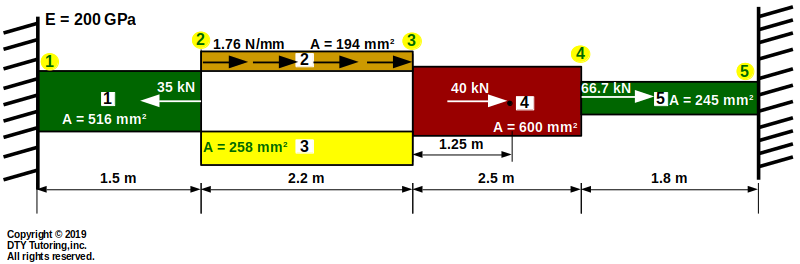StructuralAnalysisAxialEx3solution.pdf

## Displacement, Reaction and Member Forces in a Truss (Matrix Displacement Method,MDM)

7. Degrees of Freedom (DOF) for a truss member
8.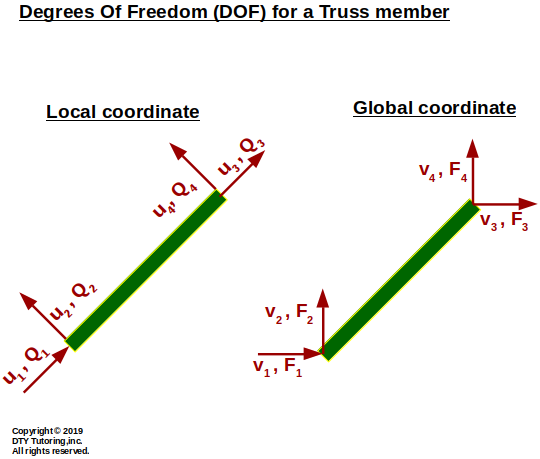9. Finding displacement, reaction and member forces using MDM method example 1 (Truss)
10. Find the displacements, member forces and reactions for this truss.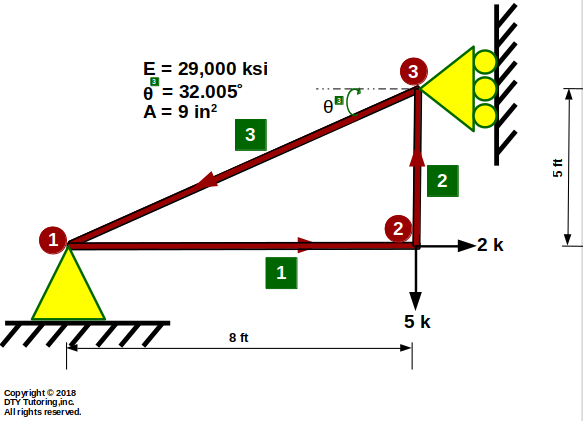StructuralAnalysisTrussEx1solution.pdf

11. Finding displacement, reaction and member forces using MDM method example 2 (Truss)
12. Find the displacements, member forces and reactions for this truss.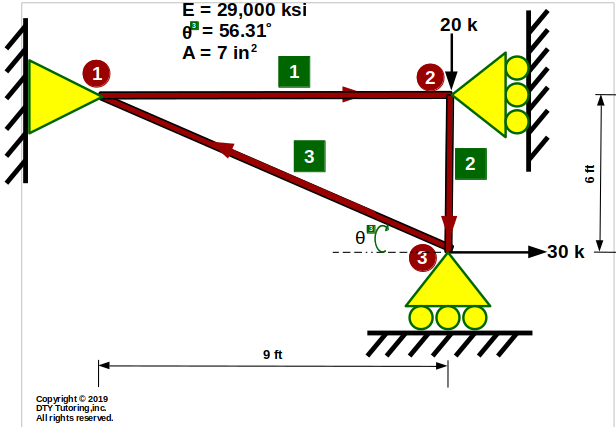StructuralAnalysisTrussEx2solution.pdf

13. Finding displacement, reaction and member forces using MDM method example 3 (Truss)
14. Find the displacements, member forces and reactions for this truss.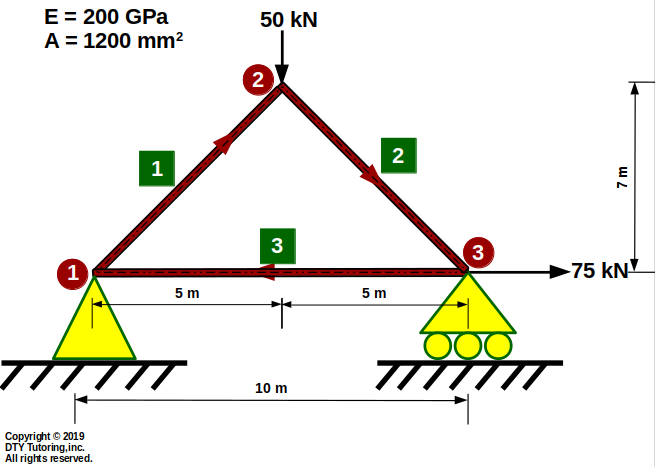StructuralAnalysisTrussEx3solution.pdf

## Displacement, Reaction and Member Forces in a Beam (Matrix Displacement Method,MDM)

15. Degrees of Freedom (DOF) for a beam member
16.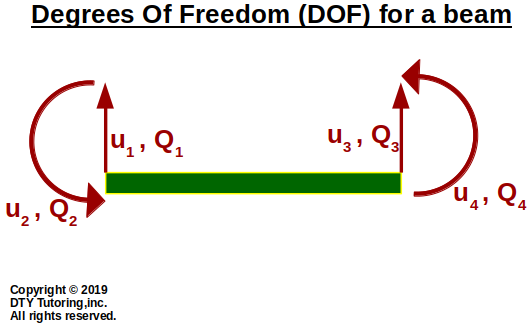17. Finding displacement, reaction and member forces using MDM method example 1(Beam)
18. Find the displacements, member forces and reactions for this beam. Then, draw the shear and moment diagrams.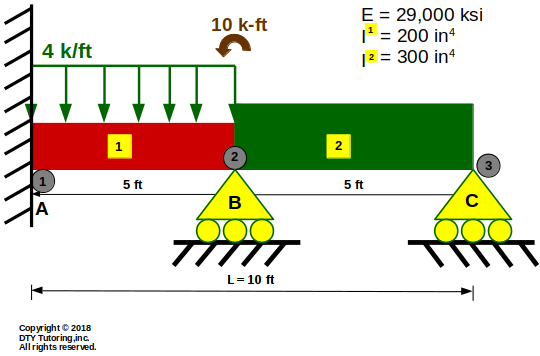StructuralAnalysisBeamEx1solution.pdf

19. Finding displacement, reaction and member forces using MDM method example 2(Beam)
20. Find the displacements, member forces and reactions for this beam. Then, draw the shear and moment diagrams.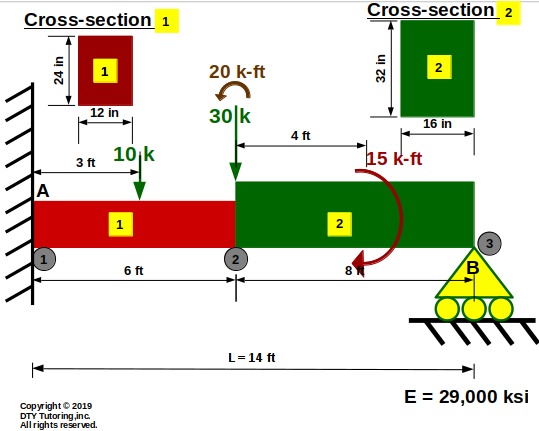StructuralAnalysisBeamEx2solution.pdf

## Displacement, Reaction and Member Forces in a Frame (Matrix Displacement Method,MDM)

21. Degrees of Freedom (DOF) for a frame member
22.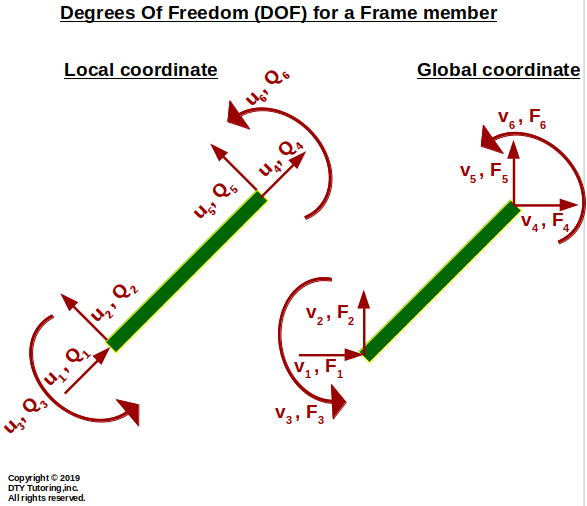23. Finding displacement, reaction and member forces using MDM method example (Frames)
24. Find the displacements, member forces and reactions for this frame shown below.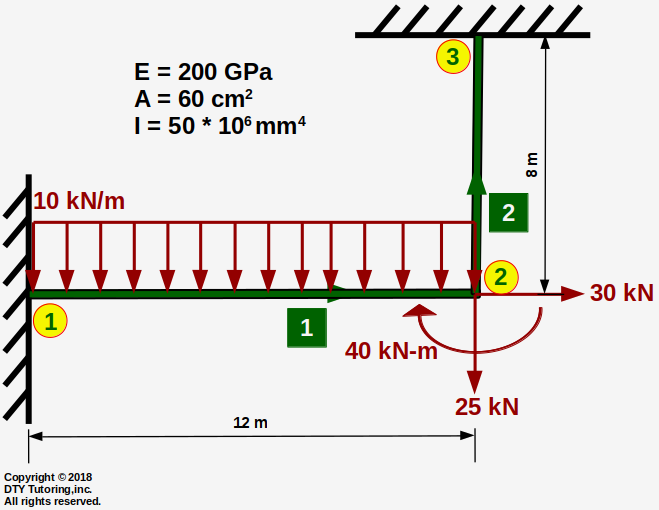StructuralAnalysisFrameEx1solution.pdf

25. Finding displacement, reaction and member forces using MDM method example (Frames)
26. Find the displacements, member forces and reactions for this frame shown below.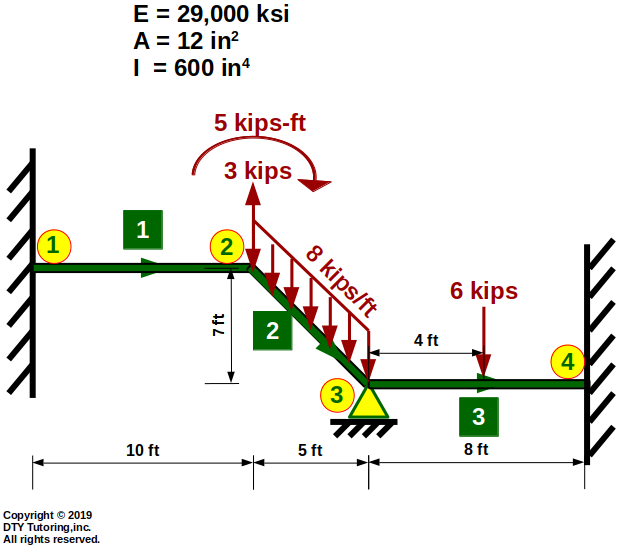StructuralAnalysisFrameEx2solution.pdf

27. Qualitative shear and moment diagrams
28. Fixed end forces derivation

### Videos

↾τxy→σx

 ↽τyx↓σy

 w(x)↧↧↧↧↧↧↧↧↧↧↧↧↧↧↧↧↧↧↧↧↧↧↧↧↧↧↧↧↧↧↧↧↧↧↧↧↧↧↧↧↧

 INQUIRE ABOUT FAQs Our contacts Our courses INFORMATION Our areas of interest Site Map About our company FOLLOW US ON